Journal DetailsFormat
Journal
First Published
09 Nov 2012
Publication timeframe
4 times per year
Languages
EnglishOpen Access

# An Analytical Study of Partially Strengthened Single End-Bearing Granular Pile Near the Top and Bottom

###### Accepted: 22 Apr 2021
Journal DetailsFormat
Journal
First Published
09 Nov 2012
Publication timeframe
4 times per year
Languages
English

Stone columns (or granular piles, GPs) are progressively being utilized for ground improvement, mostly for pliant edifice such as road mounds, oil depot, and so forth. The present analysis is done by introducing strengthening at both the ends of GP, i.e., bottom and top end so that the bulging problem will be solved and the beneficiary effect of the bearing stratum can be utilized by the bottom strengthening feature. Analysis of a single partially strengthened, at both top and bottom, end-bearing GP is presented in this article in terms of displacement affecting component for the top (DACT) of GP, percentage load transferred to the base (PLTB) of strengthened GP, and normalized shear stress (NSS). The PLTB of the strengthened GP was found to increase considerably. The NSS was found to reduce at the top end of GP and is found to be redistributed along the length of GP.

#### Keywords

Introduction

The adequate infrastructure of buildings, roads, tunnels, bridges, and other civil engineering works is a must for any country's progress. Consequently, construction is now done on places with enormously poor ground situations like soft clays, which cover a vast area in India. The rigorousness of the granular pile (GP) stops the horizontal enfolding of stones, hence aiding faster and cost-effective installation. The strengthening of the conventional GP may be achieved by (stiffened deep cement mixing) SDCM, use of geosynthetics, etc., which ultimately improves the performance of GP, owing to better mechanical properties of strengthening material used. For resolving the problem, the elastic continuum approach is applied. As the top and bottom portions are strengthened, a new matrix is created to introduce strengthening parameters for the top and bottom of GP. The parameter relative stiffness of bearing stratum, i.e., (Ebs/Ess), is defined for partially strengthened end-bearing GP, where “Ebs” and “Ess,” are, respectively, the deformation modulus of bearing stratum and surrounding soft soil. The basic mirror image method is used to obtain the solution.

This study emphasizes the single end-bearing GP with its top and bottom portions strengthened, leaving some intermediate portion as unstrengthened for economic considerations. In past research, the effect of strengthening at the top was studied for getting rid of the bulging problem, as reported earlier. Now in this study, the bottom portion of end-bearing GP is also strengthened to take advantage of the bearing stratum, and as a result, it has been found to improve the performance of GP.

The analysis is based on the following assumptions:

It is assumed that the stress-strain relationship is of linear nature.

To have a uniform distribution of load across the base of the granular pile and proper contact of the GP base with the base stratum, the base of the GP is considered as smooth by Madhav et al.  across which the load is uniformly distributed.

A linear performance is presumed to be applicable for soil, and simultaneously, it is supposed to be homogeneous and isotropic.

It is assumed that there will be no slip or yield state at the granular pile-soil interface, owing to its rough nature.

Literature Review

Description of Problem

Fig. 1 (a), (b), and (c) depict the basic plot of the problem, where a single end bearing GP having its top and bottom both portions strengthened is presented. The length of end-bearing GP is taken as “Lg” while its diameter as “Dg”= (2a). GP is assumed to be subjected to an axial load “F”. “Fb” is considered the load transferred to the base of the strengthened GP. Fig. 1 (a) shows that the top portion of single end-bearing GP is strengthened upto some definite length “Lt,” and similarly bottom portion of GP is also strengthened for a length “Lb”. A parameter “ηt” (=Lt/Lg) is chosen to show the strengthening phenomenon at the top portion of the GP. At the same time, “ηb” (=Lb/Lg) denotes the strengthening effect taken at the bottom end of the GP, leaving some portion at the middle to be unstrengthened. “Etsp,” “Ebsp,” “Emusp,” “Ess” and “Ebs” are, respectively, the modulus of deformation, of the granular pile in the top strengthened portion, bottom strengthened portion, middle un-strengthened portion, the surrounding soft soil, and the bearing stratum. The Poisson's ratio of surrounding soft soil and bearing stratum are, respectively, represented by “νss” and “νbs”. The relative stiffness of GP and bearing stratum are, respectively, defined - Kp= Emusp/Ess, i.e., the ratio of modulus of deformation of the middle portion of the GP to that of the surrounding soft soil and Kbs= Ebs/Ess, i.e., the ratio of modulus of deformation of bearing stratum to that of the surrounding soft soil. For taking the degree of strengthening into account for the top and bottom portion of the GP, respectively, the parameters “χt” and “χb” were introduced. “χt” is the factor by which the “Kp,” of the unstrengthened portion of GP, is multiplied to get the “Kp,” of the top strengthened portion of the GP and similarly, “χb” is the factor by which the “Kp”, of the unstrengthened portion of GP is multiplied to get the “Kp,” of the bottom, strengthened portion of the GP. The value of χt and χb both is ≥ 1, being unity for the unstrengthened condition of GP and greater than unity for the strengthened condition of GP. The relative length of GP is defined as Lg/Dg, i.e., ratio of length and diameter of the GP. zg is taken as the depth of an element from the top of the GP, and z*g=zg/Lg is defined as the normalized depth.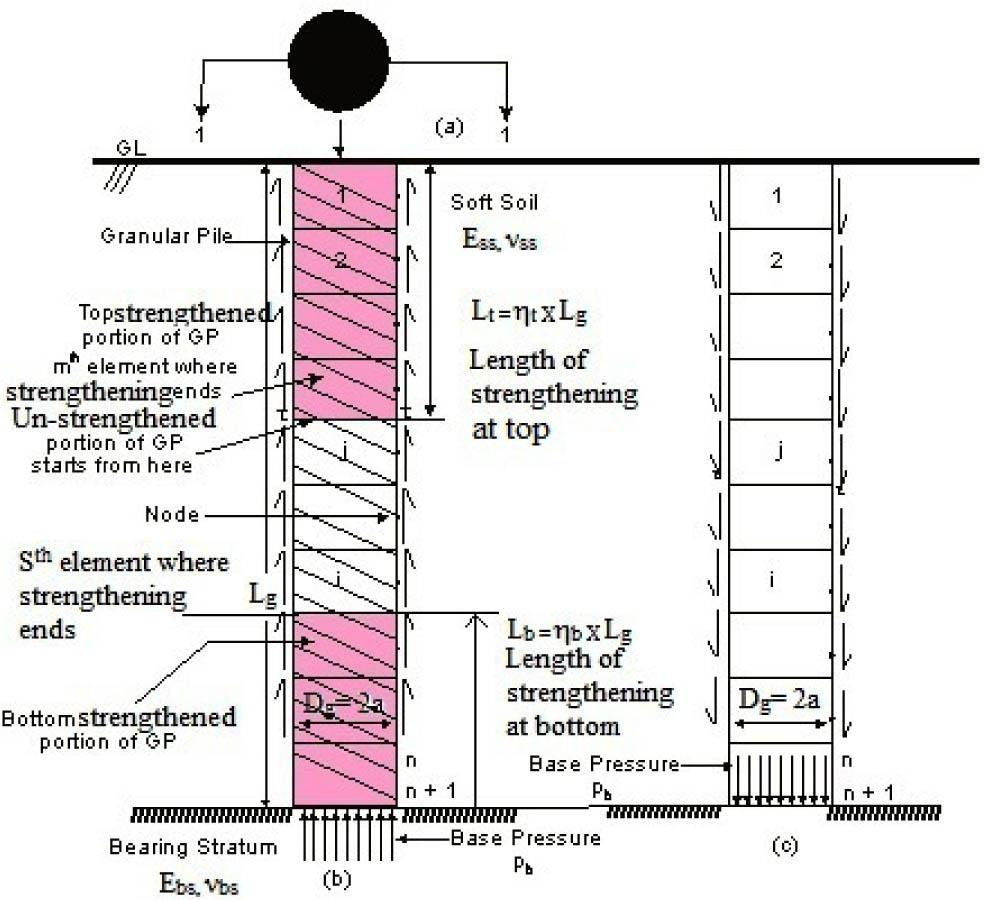(a) Plan of strengthened end bearing GP (b) Sectional elevation at 1–1 of a single strengthened end bearing GP, subjected to load F, revealing strengthening effect. (c) Problem description sketch, development of interfacial shear stresses on soil due to a single strengthened end-bearing GP.

The basic mirror image technique, as shown in Fig. 2, is applied to obtain the solution with the simultaneous imposition of elastic continuum approach. Following section deals with the method used for analysis.

Soil Displacements

For carrying out the analysis, the basic discretization and the integration scheme suggested by Mindlin  is used. So, the partially strengthened GP is discretized into “n” cylindrical elements. GP is assumed to be acted upon by shear stresses, τ, while τ*E =τ(πDgLg)/F is the NSS. It was considered that a uniform pressure “pb” is developed across the base of the GP. For the sake of analysis, nodes were considered at the periphery (at the center of each discretized element) and center of the base of GP. The soil displacements of the nodes were evaluated based on the influence of the elemental shear stresses. Thus, soil displacement equations for a GP which is end-bearing, are given by Mattes and Poulos  as described by Eq. (1). ${ρsd}={SsdDg}=[[IFCsd]−ψ[IFCsdim]]{τEss}$ \left\{ {{{\rm{\rho }}^{{\rm{sd}}}}} \right\} = \left\{ {{{{{\rm{S}}^{{\rm{sd}}}}} \over {{{\rm{D}}_{\rm{g}}}}}} \right\} = \left[ {\left[ {{\rm{IF}}{{\rm{C}}^{{\rm{sd}}}}} \right] - {\rm{\psi }}\left[ {{\rm{IF}}{{\rm{C}}^{{\rm{sdim}}}}} \right]} \right]\left\{ {{{\rm{\tau }} \over {{{\rm{E}}_{{\rm{ss}}}}}}} \right\} where {Ssd} and {ρsd} in Eq. (1) are, respectively, the soil displacement and normalized soil displacement vectors each of size “n,” while {τ/Ess} is a column vector of size (n+1). In order to account for the effect of bearing stratum, the mirror image approximation Poulos and Mattes  is applied. For considering the effect of the mirror image elements, a non-dimensional parameter, ψ, is chosen, which takes into account the compressibility of the base and its value lying between 0 and 1. The exact value of ψ is 0 for floating GP and 1 for end-bearing GP. The impact of the elements of the mirror image is taken as, ψ, times the influence of shear stresses on the real elements in the reverse direction. In Eq. (1), [IFCsd] denotes the soil displacement influence coefficients, and [IFCsdim] represents the soil displacement influence coefficients due to image elements, both are square matrices each of size “n”.

Granular Pile Displacements

Following analysis is carried out to obtain the GP displacements for end bearing GP resting on a relatively stiff bearing stratum. Boussinesqs’ equation is used to obtain the displacement of a rigid circular disc on a semi-infinite mass. So, by using Boussinesqs’ equation, displacement of the base of a GP resting on a bearing stratum of some finite compressibility is approximated by $ρbp=sbpDg=pb(1−νbs2)π/4Ebs$ {\rm{\rho }}_{\rm{b}}^{\rm{p}} = {{{\rm{s}}_{\rm{b}}^{\rm{p}}} \over {{{\rm{D}}_{\rm{g}}}}} = {{{{\rm{p}}_{\rm{b}}}\left( {1 - {\rm{\nu }}_{{\rm{bs}}}^2} \right){\rm{\pi }}/4} \over {{{\rm{E}}_{{\rm{bs}}}}}} In order to express the base pressure in terms of shear stresses, as given by Eq.(3), equilibrium equation is used as under, $pb=FπDg2/4−4(Lg/Dg)n∑j=1j=nτj$ {{\rm{p}}_{\rm{b}}} = {{\rm{F}} \over {{\rm{\pi D}}_{\rm{g}}^2/4}} - {{4\left( {{{\rm{L}}_{\rm{g}}}/{{\rm{D}}_{\rm{g}}}} \right)} \over {\rm{n}}}\sum\nolimits_{{\rm{j}} = 1}^{{\rm{j}} = {\rm{n}}} {{\tau _{\rm{j}}}} Hence, using the applied load and mobilized shear stresses, the normalized settlement of the base (using Eq.–s (2) and (3)) can be expressed as $ρb=[FEssπDg2/4−4(Lg/Dg)n∑j=1j=nτjEss]×π(1−νbs2)4(Ebs/Ess)$ {{\rm{\rho }}_{\rm{b}}} = \left[ {{{\rm{F}} \over {{{\rm{E}}_{{\rm{ss}}}}{\rm{\pi D}}_{\rm{g}}^2/4}} - {{4\left( {{{\rm{L}}_{\rm{g}}}/{{\rm{D}}_{\rm{g}}}} \right)} \over {\rm{n}}}\sum\nolimits_{{\rm{j}} = 1}^{{\rm{j}} = {\rm{n}}} {{{{\tau _{\rm{j}}}} \over {{{\rm{E}}_{{\rm{ss}}}}}}} } \right] \times {{{\rm{\pi }}\left( {1 - {\rm{\nu }}_{{\rm{bs}}}^2} \right)} \over {4\left( {{{\rm{E}}_{{\rm{bs}}}}/{{\rm{E}}_{{\rm{ss}}}}} \right)}} To evaluate the displacement of any ith element lying in the bottom strengthened portion of the GP, where s≤i≤n, it is assumed that bottom strengthening is done from the bottom nth element to the Sth element as shown in Fig. 1 (b). The displacement of the base is added with the displacement of the element due to the axial stress acting on it as given by $ρip=ρbp+∑j=nj=i−1σjEbsp(Δz/Dg)+σiEbsp(Δz/2Dg)$ {\rm{\rho }}_{\rm{i}}^{\rm{p}} = {\rm{\rho }}_{\rm{b}}^{\rm{p}} + \sum\nolimits_{{\rm{j}} = {\rm{n}}}^{{\rm{j}} = {\rm{i}} - 1} {{{{{\rm{\sigma }}_{\rm{j}}}} \over {{{\rm{E}}_{{\rm{bsp}}}}}}} \left( {\Delta {\rm{z}}/{{\rm{D}}_{\rm{g}}}} \right) + {{{{\rm{\sigma }}_{\rm{i}}}} \over {{{\rm{E}}_{{\rm{bsp}}}}}}\left( {\Delta {\rm{z}}/2{{\rm{D}}_{\rm{g}}}} \right) where σj/Ebsp, is axial strain of the jth element, σi/Ebsp, is axial strain of the ith element, and Δz is the length of element.

In the above analysis, in order to maintain the compatibility of displacements at the junction of strengthened and unstrengthened bottom portion of GP, due consideration is observed. As shown in Fig. 1 (b), the bottom strengthening is carried out till the end of the Sth element from the bottom of the GP. The displacement at the top of the Sth element or bottom of the (S+1)th element, i.e., interface of bottom strengthened and an unstrengthened portion of GP, is as given below: $ρbottom interfacep=ρbp+∑j=nj=SσjEbsp(Δz/Dg)$ {\rm{\rho }}_{{\rm{bottom}}\,{\rm{interface}}}^{\rm{p}} = {\rm{\rho }}_{\rm{b}}^{\rm{p}} + \sum\nolimits_{{\rm{j}} = {\rm{n}}}^{{\rm{j}} = {\rm{S}}} {{{{{\rm{\sigma }}_{\rm{j}}}} \over {{{\rm{E}}_{{\rm{bsp}}}}}}} \left( {\Delta {\rm{z}}/{{\rm{D}}_{\rm{g}}}} \right) To satisfy the compatibility at the interface between the bottom strengthened and an unstrengthened portion of GP, the displacement of the bottom end of the (S+1)th element of the unstrengthened portion is taken as the displacement of top of the Sth element of strengthened portion of GP. Hence, the displacement of (S+1)th element is evaluated as $ρi=S+1p=ρbp+∑j=nj=SσjEbsp(Δz/Dg)+σiEmusp(Δz/2Dg)$ {\rm{\rho }}_{{\rm{i = S + 1}}}^{\rm{p}} = {\rm{\rho }}_{\rm{b}}^{\rm{p}} + \sum\nolimits_{{\rm{j}} = {\rm{n}}}^{{\rm{j}} = {\rm{S}}} {{{{{\rm{\sigma }}_{\rm{j}}}} \over {{{\rm{E}}_{{\rm{bsp}}}}}}} \left( {\Delta {\rm{z}}/{{\rm{D}}_{\rm{g}}}} \right) + {{{{\rm{\sigma }}_{\rm{i}}}} \over {{{\rm{E}}_{{\rm{musp}}}}}}\left( {\Delta {\rm{z}}/2{{\rm{D}}_{\rm{g}}}} \right) The displacement of any ith element lying in the middle unstrengthened portion of the GP, i.e., m≤i≤S, is given by $ρi middlep=ρbp+∑j=nj=SσjEbsp(Δz/Dg)++∑j=S+1j=i−1σjEmusp(ΔzDg)+σiEmusp(Δz/2Dg)$ \matrix{{{\rm{\rho }}_{{\rm{i}}\,{\rm{middle}}}^{\rm{p}} = {\rm{\rho }}_{\rm{b}}^{\rm{p}} + \sum\nolimits_{{\rm{j}} = {\rm{n}}}^{{\rm{j}} = {\rm{S}}} {{{{{\rm{\sigma }}_{\rm{j}}}} \over {{{\rm{E}}_{{\rm{bsp}}}}}}} \left( {\Delta {\rm{z}}/{{\rm{D}}_{\rm{g}}}} \right) + } \hfill \cr { + \sum\nolimits_{{\rm{j}} = {\rm{S}} + 1}^{{\rm{j}} = {\rm{i}} - 1} {{{{{\rm{\sigma }}_{\rm{j}}}} \over {{{\rm{E}}_{{\rm{musp}}}}}}} \left( {{{\Delta {\rm{z}}} \over {{{\rm{D}}_{\rm{g}}}}}} \right) + {{{{\rm{\sigma }}_{\rm{i}}}} \over {{{\rm{E}}_{{\rm{musp}}}}}}\left( {\Delta {\rm{z}}/2{{\rm{D}}_{\rm{g}}}} \right)} \hfill \cr } In order to satisfy the compatibility at top interface between strengthened and unstrengthened portion of GP, the displacement of the bottom end of the mth element of strengthened portion is taken as the displacement of the top of the (m+1)th element of unstrengthened portion of GP. Hence the displacement of mth element is evaluated as $ρtop interface, i=mp=ρbp+∑j=nj=SσjEbsp(Δz/Dg)+∑j=S+1j=mσjEmsup(Δz/Dg)$ {\rm{\rho }}_{{\rm{top}}\,\,{\rm{interface}},\,{\rm{i}} = {\rm{m}}}^{\rm{p}} = {\rm{\rho }}_{\rm{b}}^{\rm{p}} + \sum\nolimits_{{\rm{j}} = {\rm{n}}}^{{\rm{j}} = {\rm{S}}} {{{{{\rm{\sigma }}_{\rm{j}}}} \over {{{\rm{E}}_{{\rm{bsp}}}}}}} \left( {\Delta {\rm{z}}/{{\rm{D}}_{\rm{g}}}} \right) + \sum\nolimits_{{\rm{j}} = {\rm{S}} + 1}^{{\rm{j}} = {\rm{m}}} {{{{{\rm{\sigma }}_{\rm{j}}}} \over {{{\rm{E}}_{{\rm{msup}}}}}}} \left( {\Delta {\rm{z}}/{{\rm{D}}_{\rm{g}}}} \right) In order to find out the displacement of any ith element lying in the top strengthened portion of the GP, i.e., 1≤i≤m, the following equation is utilized. $ρ1≤i≤mp=ρbp+∑j=nj=SσjEbsp(Δz/Dg)+∑j=S+1j=mσjEmsup(Δz/Dg)+∑j=mj=i−1σjEtsp(Δz/Dg)+σiEtsp(Δz/2Dg)$ \matrix{{{\rm{\rho }}_{{\rm{1}} \le {\rm{i}} \le {\rm{m}}}^{\rm{p}}} \hfill & = \hfill & {{\rm{\rho }}_{\rm{b}}^{\rm{p}} + \sum\nolimits_{{\rm{j}} = {\rm{n}}}^{{\rm{j}} = {\rm{S}}} {{{{{\rm{\sigma }}_{\rm{j}}}} \over {{{\rm{E}}_{{\rm{bsp}}}}}}} \left( {\Delta {\rm{z}}/{{\rm{D}}_{\rm{g}}}} \right) + \sum\nolimits_{{\rm{j}} = {\rm{S}} + 1}^{{\rm{j}} = {\rm{m}}} {{{{{\rm{\sigma }}_{\rm{j}}}} \over {{{\rm{E}}_{{\rm{msup}}}}}}} \left( {\Delta {\rm{z}}/{{\rm{D}}_{\rm{g}}}} \right)} \hfill \cr {} \hfill & {} \hfill & { + \sum\nolimits_{{\rm{j}} = {\rm{m}}}^{{\rm{j}} = {\rm{i}} - 1} {{{{{\rm{\sigma }}_{\rm{j}}}} \over {{{\rm{E}}_{{\rm{tsp}}}}}}} \left( {\Delta {\rm{z}}/{{\rm{D}}_{\rm{g}}}} \right) + {{{{\rm{\sigma }}_{\rm{i}}}} \over {{{\rm{E}}_{{\rm{tsp}}}}}}\left( {\Delta {\rm{z}}/2{{\rm{D}}_{\rm{g}}}} \right)} \hfill \cr } Using the matrix form, the above set of displacement equations is expressed as ${ρp}=ρb{1}+[MAT1]{σEss}$ \left\{ {{\rho ^p}} \right\} = {\rho _b}\left\{ 1 \right\} + \left[ {MA{T_1}} \right]\left\{ {{\sigma \over {{E_{ss}}}}} \right\} In Eq. (11), [MAT1] is an upper triangular matrix of size (n×n) obtained by incorporating the strengthening parameters for top and bottom of the granular pile in Eq. (5) and is given by Eq. (12) $[MAT1]=(LgDg)nKp[⋅0.5χt1χt1χt1−−1χb1χb1χb00.5χt1χt1−−1χb1χb1χb000.5χt1−−1χb1χb1χb0000.5−−1χb1χb1χb⋅⋅⋅⋅⋅⋅⋅⋅⋅00000.511χb1χb1χb000000.51χb1χb1χb⋅⋅⋅⋅⋅⋅⋅⋅⋅000000000.5χb]$ \left[ {{\rm{MA}}{{\rm{T}}_1}} \right] = {{\left( {{{{{\rm{L}}_{\rm{g}}}} \over {{{\rm{D}}_{\rm{g}}}}}} \right)} \over {{\rm{n}}{{\rm{K}}_{\rm{p}}}}}\left[ { \cdot \matrix{ {{{0.5} \over {{\chi _t}}}} & {{1 \over {{\chi _t}}}} & {{1 \over {{\chi _t}}}} & 1 & - & - & {{1 \over {{\chi _b}}}} & {{1 \over {{\chi _b}}}} & {{1 \over {{\chi _b}}}} \cr 0 & {{{0.5} \over {{\chi _t}}}} & {{1 \over {{\chi _t}}}} & 1 & - & - & {{1 \over {{\chi _b}}}} & {{1 \over {{\chi _b}}}} & {{1 \over {{\chi _b}}}} \cr 0 & 0 & {{{0.5} \over {{\chi _t}}}} & 1 & - & - & {{1 \over {{\chi _b}}}} & {{1 \over {{\chi _b}}}} & {{1 \over {{\chi _b}}}} \cr 0 & 0 & 0 & {0.5} & - & - & {{1 \over {{\chi _b}}}} & {{1 \over {{\chi _b}}}} & {{1 \over {{\chi _b}}}} \cr \cdot & \cdot & \cdot & \cdot & \cdot & \cdot & \cdot & \cdot & \cdot \cr 0 & 0 & 0 & 0 & {0.5} & 1 & {{1 \over {{\chi _b}}}} & {{1 \over {{\chi _b}}}} & {{1 \over {{\chi _b}}}} \cr 0 & 0 & 0 & 0 & 0 & {0.5} & {{1 \over {{\chi _b}}}} & {{1 \over {{\chi _b}}}} & {{1 \over {{\chi _b}}}} \cr \cdot & \cdot & \cdot & \cdot & \cdot & \cdot & \cdot & \cdot & \cdot \cr 0 & 0 & 0 & 0 & 0 & 0 & 0 & 0 & {{{0.5} \over {{\chi _b}}}} \cr } } \right] Further using Eq. (4) for replacing the base displacement, Eq. (11) can be rewritten as ${ρp}=F(1−νbs2)(Ebs/Ess)Dg2Ess{1}−π(Lg/Dg)(1−νbs2)n(Ebs/Ess){τEss}+[MAT1]{σEss}$ \left\{ {{{\rm{\rho }}^{\rm{p}}}} \right\} = {{{\rm{F}}\left( {1 - {\rm{\nu }}_{{\rm{bs}}}^2} \right)} \over {\left( {{{\rm{E}}_{{\rm{bs}}}}/{{\rm{E}}_{{\rm{ss}}}}} \right){\rm{D}}_{\rm{g}}^2{{\rm{E}}_{{\rm{ss}}}}}}\left\{ 1 \right\} - {{{\rm{\pi }}\left( {{{\rm{L}}_{\rm{g}}}/{{\rm{D}}_{\rm{g}}}} \right)\left( {1 - {\rm{\nu }}_{{\rm{bs}}}^2} \right)} \over {{\rm{n}}\left( {{{\rm{E}}_{{\rm{bs}}}}/{{\rm{E}}_{{\rm{ss}}}}} \right)}}\left[ 1 \right]\left\{ {{{\rm{\tau }} \over {{{\rm{E}}_{{\rm{ss}}}}}}} \right\} + \left[ {{\rm{MA}}{{\rm{T}}_1}} \right]\left\{ {{{\rm{\sigma }} \over {{{\rm{E}}_{{\rm{ss}}}}}}} \right\} Here, {1} and  are, respectively, unit column vector and unit square matrix each of size “n.” Based on equilibrium relationship, the shear stresses in the shaft and axial stresses of elements are interconnected by the following equation: $σi=F(πDg2/4)−∑j=1j=i−1−4τjLgnDg−2τiLgnDg$ {{\rm{\sigma }}_{\rm{i}}} = {{\rm{F}} \over {\left( {{\rm{\pi D}}_{\rm{g}}^2/4} \right)}} - \sum\nolimits_{{\rm{j}} = 1}^{{\rm{j}} = {\rm{i}} - 1} { - {{{\rm{4}}{{\rm{\tau }}_{\rm{j}}}{{\rm{L}}_{\rm{g}}}} \over {{\rm{n}}{{\rm{D}}_{\rm{g}}}}} - {{{\rm{2}}{{\rm{\tau }}_{\rm{i}}}{{\rm{L}}_{\rm{g}}}} \over {{\rm{n}}{{\rm{D}}_{\rm{g}}}}}} Using matrix form, the above equation may be written for elements i = 1 to “n” as ${σEss}=F(πDg2/4)Ess−4(Lg/Dg)n[MAT2]{τEss}$ \left\{ {{{\rm{\sigma }} \over {{{\rm{E}}_{{\rm{ss}}}}}}} \right\} = {{\rm{F}} \over {\left( {{\rm{\pi D}}_{\rm{g}}^2{\rm{/4}}} \right){{\rm{E}}_{{\rm{ss}}}}}} - {{{\rm{4}}\left( {{{\rm{L}}_{\rm{g}}}/{{\rm{D}}_{\rm{g}}}} \right)} \over {\rm{n}}}\left[ {{\rm{MA}}{{\rm{T}}_2}} \right]\left\{ {{{\rm{\tau }} \over {{{\rm{E}}_{{\rm{ss}}}}}}} \right\} where [MAT2] is a lower triangular matrix having the diagonal and off-diagonal terms as 0.5 and 1.0, respectively, and is of size “n.”

The final form of displacement equations for elements i = 1 to “n” in terms of shaft shear stresses Eq. (15) is obtained in Eq. (16), using the relationship between axial stresses and shaft shear stresses: ${ρp}={Y}+[MAT]{τEss}$ \left\{ {{\rho ^p}} \right\} = \left\{ Y \right\} + \left[ {MAT} \right]\left\{ {{\tau \over {{E_{ss}}}}} \right\} where ${Y}=F(1−υbs2)(Ebs/Ess)Dg2Ess{1}+F(πDg2/4)Ess[MAT1]{1}[MAT]=−4(Lg/Dg)n[MAT1][MAT2]−π(Lg/Dg)(1−νbs2)n(Ebs/Ess)$ \matrix{ {\left\{ {\rm{Y}} \right\}} & = & {{{{\rm{F}}\left( {1 - {\rm{\upsilon }}_{{\rm{bs}}}^2} \right)} \over {\left( {{{\rm{E}}_{{\rm{bs}}}}/{{\rm{E}}_{{\rm{ss}}}}} \right){\rm{D}}_{\rm{g}}^2{{\rm{E}}_{{\rm{ss}}}}}}\left\{ 1 \right\} + {{\rm{F}} \over {\left( {{\rm{\pi D}}_{\rm{g}}^2/4} \right){{\rm{E}}_{{\rm{ss}}}}}}\left[ {{\rm{MA}}{{\rm{T}}_1}} \right]\left\{ 1 \right\}} \cr {\left[ {{\rm{MAT}}} \right]} & = & { - {{4\left( {{{\rm{L}}_{\rm{g}}}/{{\rm{D}}_{\rm{g}}}} \right)} \over {\rm{n}}}\left[ {{\rm{MA}}{{\rm{T}}_{\rm{1}}}} \right]\left[ {{\rm{MA}}{{\rm{T}}_2}} \right] - {{{\rm{\pi }}\left( {{{\rm{L}}_{\rm{g}}}/{{\rm{D}}_{\rm{g}}}} \right)\left( {1 - {\rm{\nu }}_{{\rm{bs}}}^2} \right)} \over {{\rm{n}}\left( {{{\rm{E}}_{{\rm{bs}}}}/{{\rm{E}}_{{\rm{ss}}}}} \right)}}\left[ 1 \right]} \cr }

Soil and Strengthened GP Displacement Compatibility

Solutions are obtained in terms of interface shear stresses and base pressure, after applying the compatibility of displacements of the GP and the soil as described below,

The interface shear stresses are evaluated for granular pile resting on stiff bearing stratum using Eq.–s (1) and (16) as ${τEss}=[[IFCsd]−ψ[IFCsdim]−[MAT]]−1{Y}$ \left\{ {{\tau \over {{E_{ss}}}}} \right\} = {\left[ {\left[ {IF{C^{sd}}} \right] - \psi \left[ {IF{C^{sdim}}} \right] - \left[ {MAT} \right]} \right]^{ - 1}}\left\{ Y \right\} The iterative technique (Poulos and Mattes ) is used, for estimating ψ. Eq.–s (18) and (3) are solved to evaluate the “n” unknown shear stresses, τ, and base pressure, pb, by allocating some initial value to, ψ. After getting the solution for that initial allocated value of ψ, a more accurate value is evaluated for ψ, by considering the compatibility between displacements of soil and the bearing stratum at the pile tip. At the pile tip, the soil $ρbs=sbsDg={IFCjsd−ψIFCjsdim}{τEss}=∑j=1j=n(IFCjsd−ψIFCjsdim)τjEss$ {\rm{\rho }}_{\rm{b}}^{\rm{s}} = {{{\rm{s}}_{\rm{b}}^{\rm{s}}} \over {{{\rm{D}}_{\rm{g}}}}} = \left\{ {{\rm{IFC}}_{\rm{j}}^{{\rm{sd}}} - {\rm{\psi IFC}}_{\rm{j}}^{{\rm{sdim}}}} \right\}\left\{ {{{\rm{\tau }} \over {{{\rm{E}}_{{\rm{ss}}}}}}} \right\} = {{\sum\nolimits_{{\rm{j}} = 1}^{{\rm{j}} = {\rm{n}}} {\left( {{\rm{IFC}}_{\rm{j}}^{{\rm{sd}}} - {\rm{\psi IFC}}_{\rm{j}}^{{\rm{sdim}}}} \right){{\rm{\tau }}_{\rm{j}}}} } \over {{{\rm{E}}_{{\rm{ss}}}}}} Due to shear stresses on real and imaginary elements “j,” in the above equation, $IFCjsd$ IFC_j^{sd} and $IFCjsdim$ IFC_j^{sdim } are the displacement influence coefficients for the tip, respectively. But due to symmetrical conditions, $IFCjsd=IFCjsdim$ IFC_j^{sd} = IFC_j^{sdim } . Now the new values of the parameter ψ are obtained by equating the soil displacement at the pile tip to the displacement of the base due to base stress, pb, Eq. (2) as given by $ψ=1−π(1−νbs2)pb4(Ebs/Ess)∑j=1j=nτjIFCjsd$ {\rm{\psi }} = 1 - {{{\rm{\pi }}\left( {1 - {\rm{\nu }}_{{\rm{bs}}}^2} \right){{\rm{p}}_{\rm{b}}}} \over {4\left( {{{\rm{E}}_{{\rm{bs}}}}/{{\rm{E}}_{{\rm{ss}}}}} \right)\sum\nolimits_{{\rm{j}} = 1}^{{\rm{j}} = {\rm{n}}} {{{\rm{\tau }}_{\rm{j}}}{\rm{IFC}}_{\rm{j}}^{{\rm{sd}}}} }} This process of iteration is repeated as just described again and again by solving Eq. (18) iteratively using the new value of ψ, and the process is repeated until the percentage difference between the new value of ψ and last calculated value of ψ becomes as low as 0.01%, i.e., a very high-order convergence is achieved. The normalized top displacement of a single partially strengthened GP is obtained as $ρtop=StopDg=Fπ4EssDg2DT$ {{\rm{\rho }}_{{\rm{top}}}} = {{{{\rm{S}}_{{\rm{top}}}}} \over {{{\rm{D}}_{\rm{g}}}}} = {{\rm{F}} \over {{{\rm{\pi }} \over 4}{{\rm{E}}_{{\rm{ss}}}}{\rm{D}}_{\rm{g}}^2}}{{\rm{D}}_{\rm{T}}} The top displacement of a single partially stiffened GP is obtained as $Stop=Fπ4EssDgDT$ {{\rm{S}}_{{\rm{top}}}} = {{\rm{F}} \over {{{\rm{\pi }} \over 4}{{\rm{E}}_{{\rm{ss}}}}{{\rm{D}}_{\rm{g}}}}}{{\rm{D}}_{\rm{T}}} In above Eq.–s (21) and (22), DT is the displacement affecting component for the top (DACT) of GP.

The parameters that are found affecting the above displacement analysis are enlisted:

Relative length, i.e., ratio of length and diameter of the GP, (Lg/Dg),

The relative stiffness of GP, Kp = (Emusp/Ess),

In the case of the end bearing GP, the relative stiffness of bearing stratum, Kbs = Ebs/Ess.

Poisson's ratios of the surrounding soft soil, νss and that of the base stratum, νbs.

Percentage length of strengthening from the top (PLST) of GP, ηt (=Lt/Lg).

Percentage length of strengthening from the bottom (PLSB) of GP, ηb (=Lb/Lg).

Strengthening factor for the top and bottom, χt and χb respectively.

Results and Discussion

The results for displacement effecting component for top of GP, DT, are validated with the results obtained by Poulos and Mattes  in Table 1 for an unstrengthened single end-bearing GP, by selecting the values of both the parameters viz. strengthening factor for the top and strengthening factor for the bottom as unity.

Validation of results.

Variables verified Lg/Dg Kp and Ebs/Ess Results of Poulos and Mattes  Present analysis with (χtb=1)
DACT (DT) of GP 25 100 and 10 0.54 0.539
25 100 and 100 0.51 0.508
25 100 and 1000 0.49 0.489
20 100 and 100000 0.09 0.0899

The following paragraphs deal with the results and discussion of the ongoing analysis, by making the GP partially strengthened. Results, in general, are obtained for the following ranges of the above-defined parameters

Kp = 50–400, ηt =10–40%, ηb =10–40%, χb =1–5, χt =1–5, Lg/Dg=10–40, Ebs/Ess=10–100. The Poisson's ratio values for soil and bearing stratum are taken each equal to 0.5 in the following analysis.

Fig. 3 represents the variation of displacement affecting component for the top of GP, DT, with strengthening factor for the top, χt, or strengthening factor for the bottom, χb. Both the PLST of GP and PLBT from the bottom of GP are taken as 10%, as shown in Fig. 3. As depicted in Fig. 3, in some curves, χt is taken as unity, implying that the strengthening effect is only applied at the bottom end. Hence, here the effect of variation of χb is depicted. Similarly, when χb is taken as one, it indicates that the strengthening effect is adopted at the top end of GP only, and hence its variation is shown. It can well be seen from the curves that when the strengthening effect is only provided at the bottom of GP, then the curve is almost linear, representing that this is not making considerable outcome on the parameter of displacement affecting component for the top of GP, DT. But for the case of strengthening adopted for the bottom, the displacement affecting component for the top of GP, DT, decreases as the value strengthening factor for the top, χt, increases. Besides this, it is visible that the value of displacement affecting component for the top of GP, DT, decrease with an increase in relative stiffness of GP, Kp as well as relative stiffness of bearing stratum Ebs/Ess, whatever may be the case, i.e., bottom strengthening or top strengthening. It may well be observed from the Fig. 3 that at– Lg/Dg =10, Ebs/Ess=100, χt =1, ηt=10%, Kp=50, for χb=1, 2, 3, 4, and 5, the values of displacement affecting component for top of GP, DT are, respectively, 0.1228, 0.1150, 0.1124, 0.1110, and 0.1103, thus causing a decrease in percentage of 6.3, 2.2, 1.2, and 0.6 with respect to χb =1; and at Lg/Dg =10, Ebs/Ess =100, χt =1, ηt=10%, Kp=100, for χb=1,2,3,4,5, the values of displacement affecting component for top of GP, DT are, respectively, 0.0775, 0.0733, 0.0719, 0.0712, and 0.0708, thus causing a percentage decrease of 5.4, 1.9, 0.9, and 0.5 with respect to χb=1.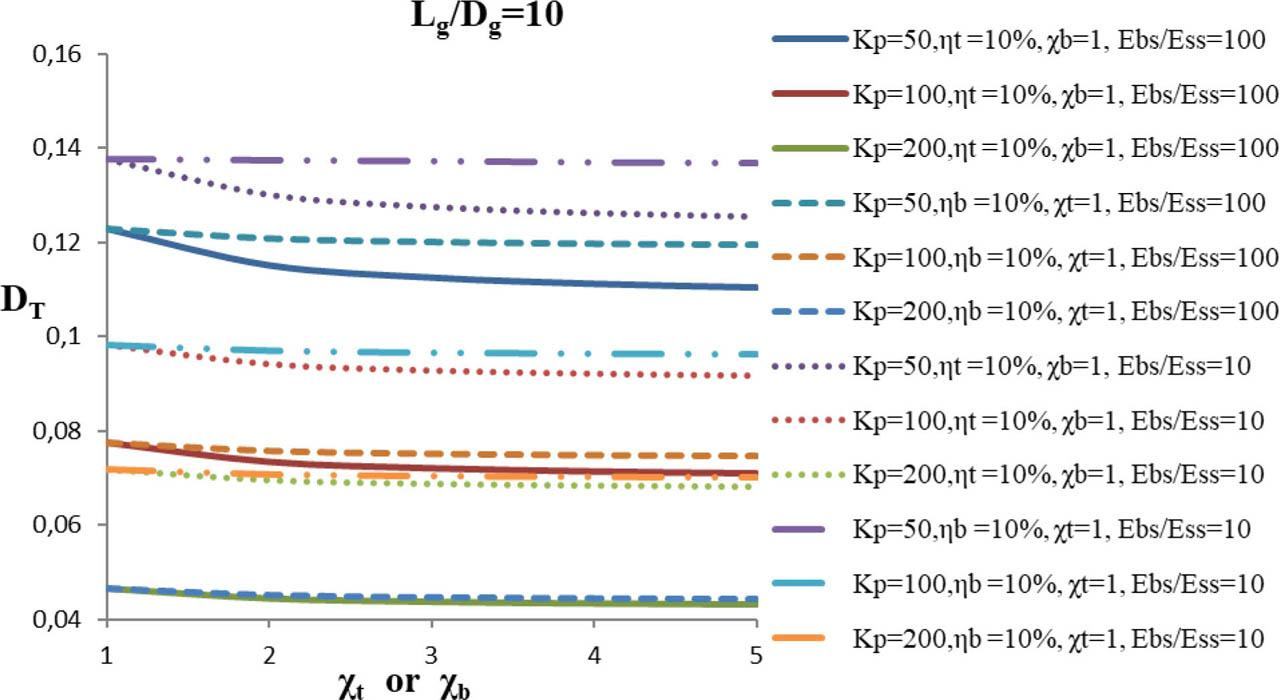Variation of displacement affecting component for the top of GP, DT, with strengthening factor for the top, χt, or strengthening factor for the bottom, χb. Impact of the relative stiffness of GP, Kp; PLST of GP, ηt; PLSB of GP, ηb; and relative stiffness of bearing stratum, Ebs/Ess, on a single partially strengthened GP(Lg/Dg=10, ηt or ηb=10%).

Fig. 4 shows the variation of displacement affecting component for the top of GP, DT, with strengthening factor for the top, χt, or strengthening factor for the bottom, χb, as explained in Fig. 3. Both the PLST of GP and PLSB of GP are taken as 20%, shown in Fig. 4. Thus, in contrast to Fig. 3, the percentage length of strengthening is increased; rest effect is as it is. Hence, it can be observed from the curves that the values of displacement affecting component for the top of GP, DT, are less than of the values in Fig. 3. For example, from observing the curves, it may well be noted that at, Lg/Dg=10, Ebs/Ess=100, χt=1, ηt=20%, Kp=100, for χb=1, 2, 3, 4, and 5, the values of displacement affecting component for top of GP, DT, are, respectively, 0.0775, 0.0684, 0.0653, 0.0637, and 0.0627, so causing a large percentage decrease of 11.7, 4.5, 2.4, and 1.5 with respect to χb=1.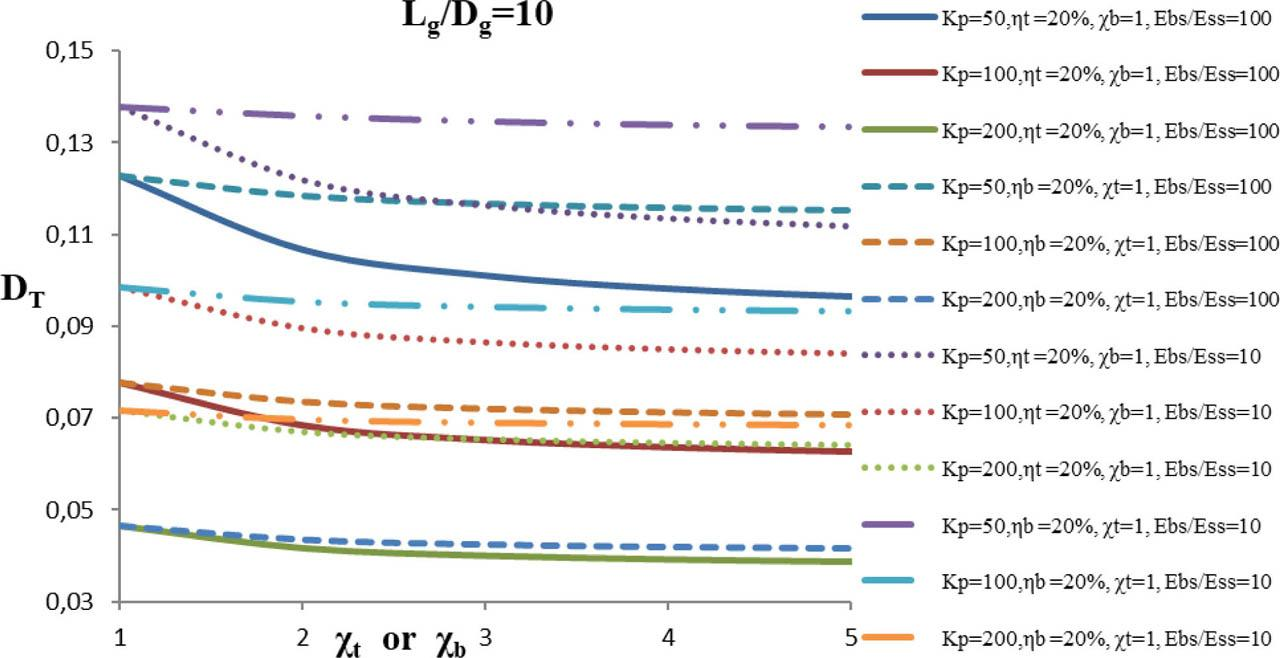Variation of displacement affecting component for the top of GP, DT, with strengthening factor for the top, χt, or strengthening factor for the bottom, χb. Impact of the relative stiffness of GP, Kp; PLST of GP, ηt; PLSB of GP, ηb; and relative stiffness of bearing stratum, Ebs/Ess, on a single partially strengthened GP (Lg/Dg=10, ηt or ηb=20%).

Fig. 5 shows the variation of displacement affecting component for the top of GP, DT, with strengthening factor for the top, χt, or strengthening factor for the bottom, χb. Both the PLST of GP and the PLSB of GP are further increased to 30% in the Fig. 5. By increasing the percentage length of strengthening, the values of displacement effecting component for the top of GP decrease more. The effect of the relative stiffness of the bearing stratum can well be seen from the Fig. 5. As shown in the Fig. 5, the value of displacement affecting component for top of GP, DT, at Lg/Dg=10, Ebs/Ess=100, χt=1, ηt=30%, Kp=100, χt=2 is 0.0869, while with Ebs/Ess=10 and rest parameters as it is, the value turns out to be 0.0638, which decreases to 26.58%.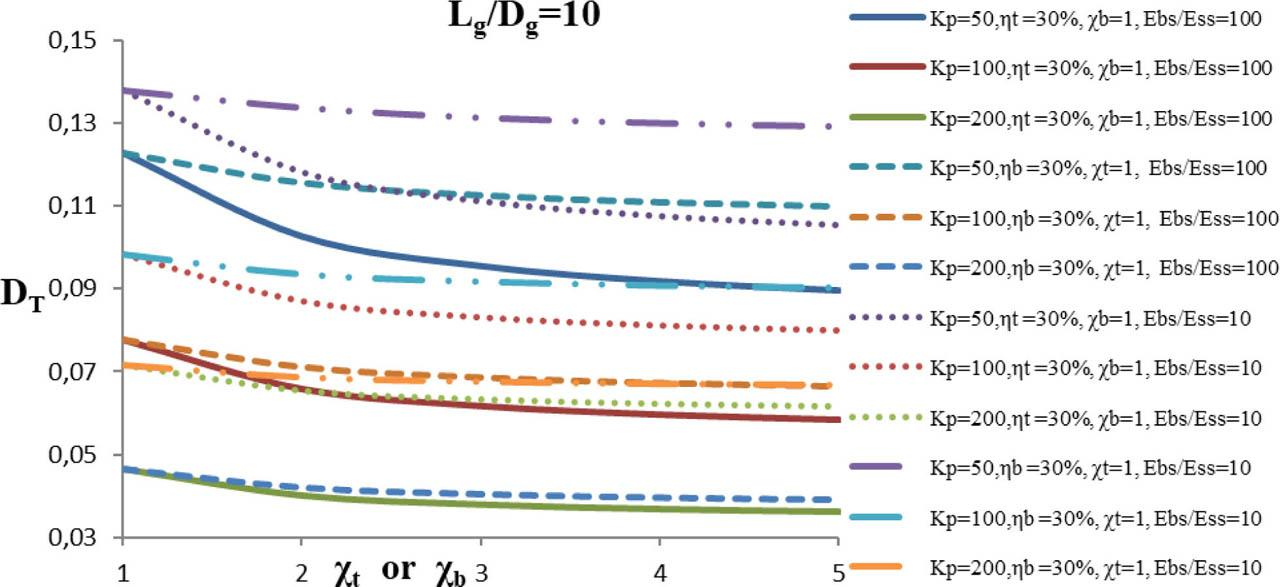Variation of displacement affecting component for the top of GP, DT, with strengthening factor for the top, χt, or strengthening factor for the bottom, χb. Impact of the relative stiffness of GP, Kp; PLST of GP, ηt; PLSB of GP, ηb; and relative stiffness of bearing stratum, Ebs/Ess, on a single partially strengthened GP (Lg/Dg=10, ηt or ηb=30%).

Fig. 6 represents the variation of displacement affecting component for the top of GP, DT, with strengthening factor for the top, χt or strengthening factor for the bottom, χb, such that χtb. Due to simultaneous dual strengthening, the values of displacement affecting component for the top of GP, DT are less than to the values shown in earlier figures. Also, it is visible from the Fig. 6 that top strengthening causes more reduction in displacement affecting component for top of GP, DT, as compared to the bottom strengthening. For example, it may be seen from the figure that at– Lg/Dg=10, Ebs/Ess=100, ηt=30%, ηb=10%, χtb=3 for Kp=50, 100, 200, and 400, the values of displacement affecting component for top of GP, DT are, respectively, 0.0925, 0.0590, 0.0359, and 0.0219, whereas at– Lg/Dg=10, Ebs/Ess=100, ηt= 10%, ηb= 30%, χtb=3 for, Kp=50, 100, 200, and 400, are respectively 0.1020, 0.0629, 0.0373, and 0.0224. Hence, it can be seen that the value of displacement affecting component for the top of GP decreases with the increase in the value of Kp.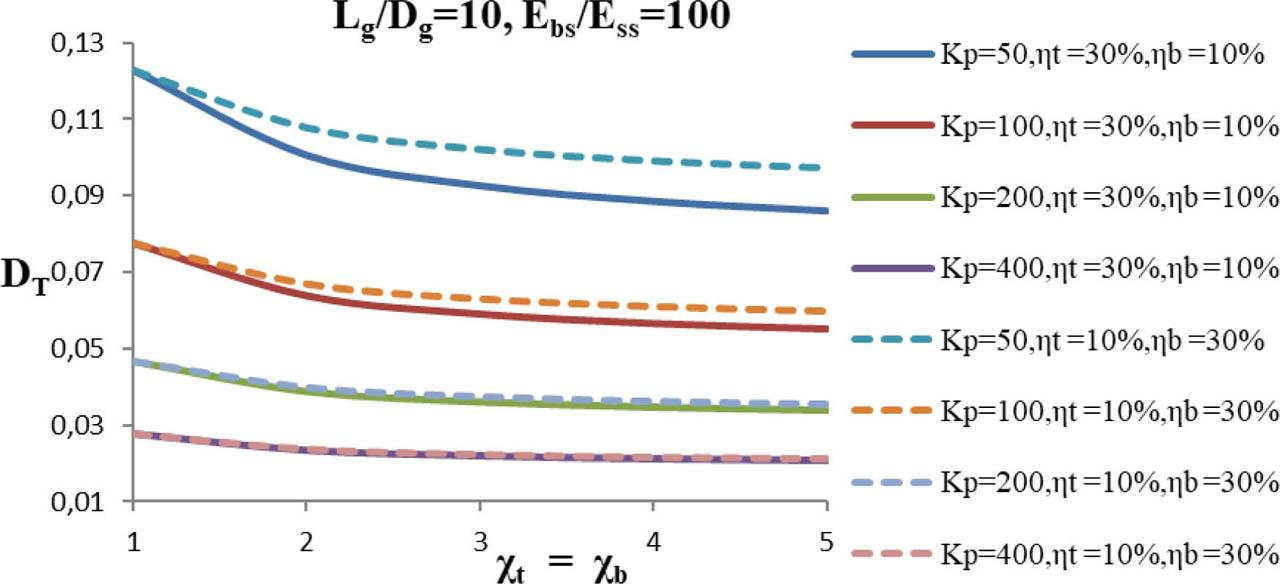Variation of displacement affecting component for the top of GP, DT, with strengthening factor for the top, χt or strengthening factor for the bottom, χb, such that χt= χb. Impact of the relative stiffness of GP, Kp; PLST of GP, ηt; PLSB of GP, ηb; on a single partially strengthened GP (Lg/Dg=10, Ebs/Ess=100).

Fig. 7 shows the variation of displacement affecting component for top of GP, DT, with strengthening factor for the top, χt or strengthening factor for the bottom, χb, such that χt= χb. In this Fig. 7, three factors vary namely relative stiffness of GP, Kp; PLST of GP- is increased further as in contrast to Fig. 6, i.e., ηt =20% or 40%, similarly PLSB of GP also increased likewise, ηb=40% and 20%. The nature of the curves is same as in previous one, with a variation in values, i.e., in this case, the values are a little lower than the previous one because of higher percentage length of strengthening. For instance, at– Lg/Dg=10, Ebs/Ess=100, ηt=40%, ηb=20%, χtb=3 for Kp= 50, 100, 200, and 400, the values of displacement effecting component for top of GP, DT, are, respectively, 0.0821, 0.0513, 0.0310, and 0.0191, whereas at– Lg/Dg=10, Ebs/Ess=100, ηt=20%, ηb=40%, χtb=3 for Kp= 50, 100, 200, and 400, are, respectively, 0.0854, 0.0523, 0.0312, and 0.0190. However in Fig. 6, the values of displacement effecting component for top of GP, DT, at– Lg/Dg=10, Ebs/Ess=100, ηt= 10%, ηb=30%, χtb=3 for Kp= 50, 100, 200, and 400, are, respectively, 0.1020, 0.0629, 0.0373, and 0.0224; hence it is clear that DT decreased with the increase in values of ηt and ηb.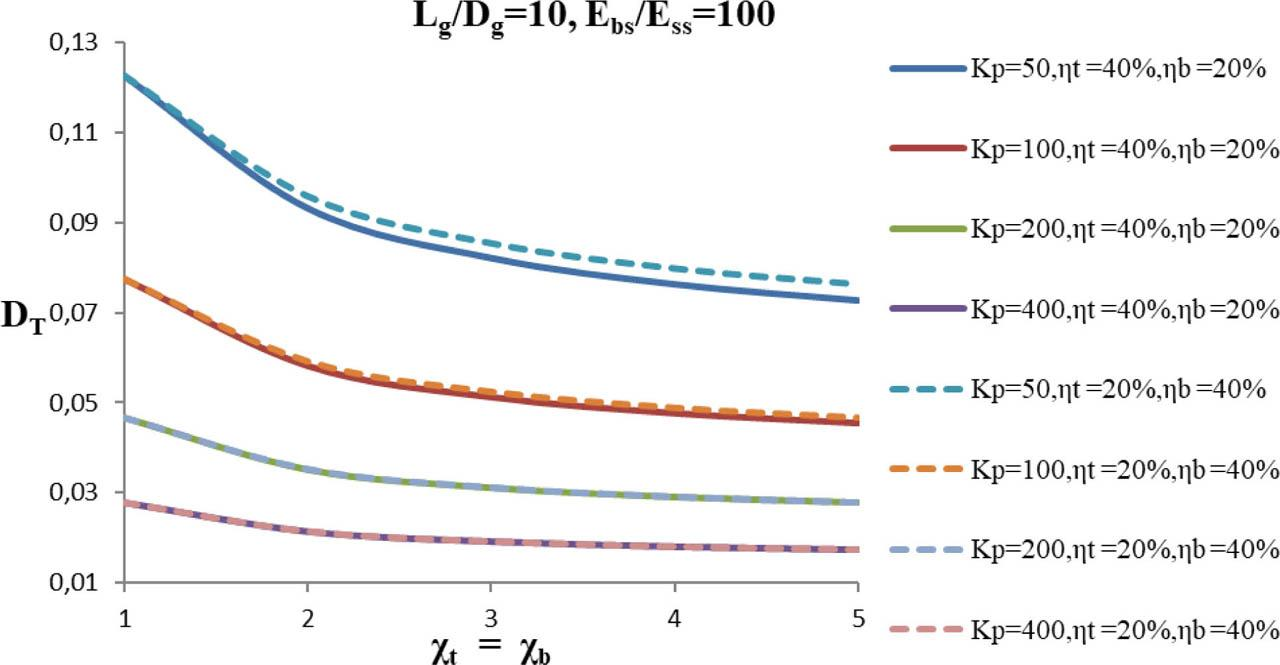Variation of displacement affecting component for the top of GP, DT, with strengthening factor for the top, χt, or strengthening factor for the bottom, χb such that χt= χb. Impact of the relative stiffness of GP, Kp; PLST of GP, ηt; PLSB of GP, ηb; on a single partially strengthened GP (Lg/Dg=10, Ebs/Ess =100).

Fig. 8 shows the variation of displacement affecting component for top of GP, DT, with strengthening factor for the top, χt, or strengthening factor for the bottom, χb, such that χtb. The variation of three parameters namely relative stiffness of GP, Kp; PLST of GP, ηt; PLSB of GP, ηb; such that ηtb=30% is depicted here. At Lg/Dg=10, Ebs/Ess=100, ηt=30%, ηb=30%, χtb=3 for Kp= 50, 100, 200, and 400, the values are, respectively, 0.0844, 0.0522, 0.0314, and 0.0192, which are somewhat closer to the values shown in Fig. 7; at– Lg/Dg=10, Ebs/Ess=100, ηt= 20%, ηb= 40%, χt= χb=3 for Kp= 50, 100, 200, and 400, are, respectively, 0.0854, 0.0523, 0.0312, and 0.0190.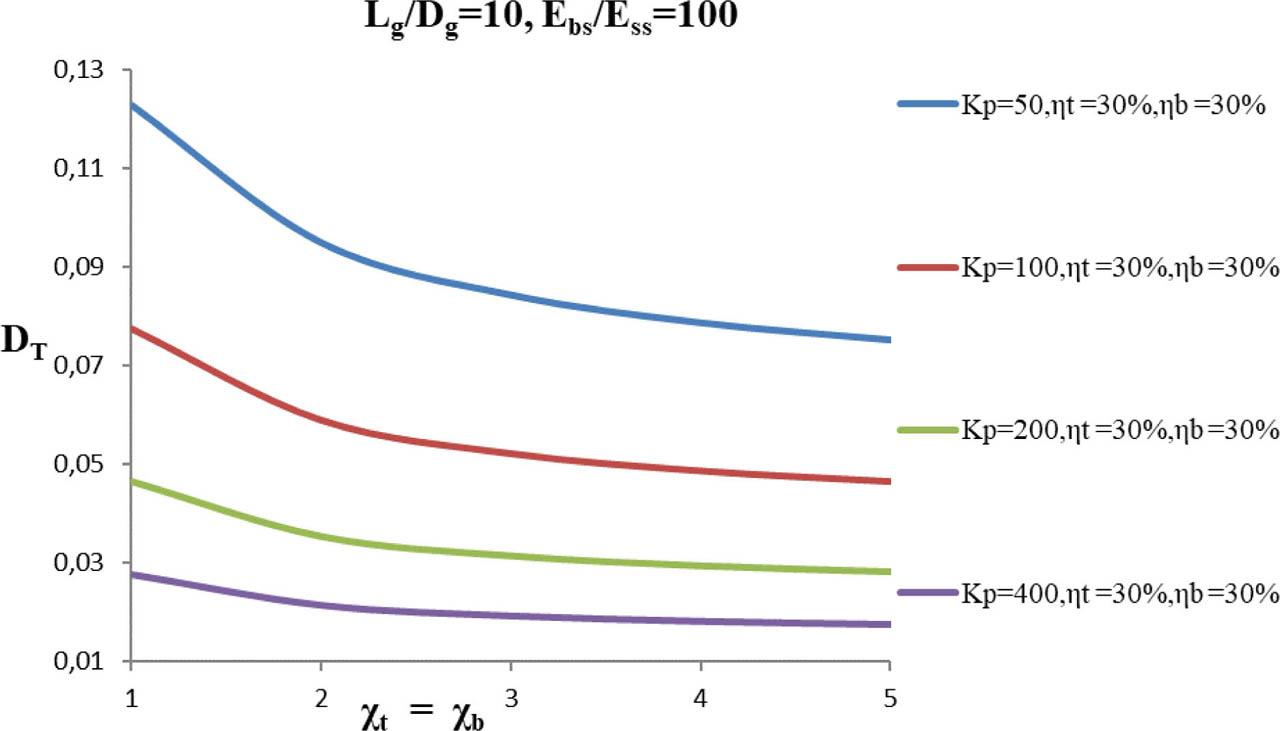Variation of displacement affecting component for the top of GP, DT, with strengthening factor for the top, χt or strengthening factor for the bottom, χb, such that χt=χb. Impact of the relative stiffness of GP, Kp; PLST of GP, ηt; PLSB of GP, ηb; on a single partially strengthened GP (Lg/Dg=10, Ebs/Ess =100)

Fig. 9 shows the variation of displacement affecting component for the top of GP, DT, with strengthening factor for the top, χt, or strengthening factor for the bottom, χb. This Fig. 9 depicts the variation of three different parameters: the relative length of GP, Lg/Dg, both the PLST of GP and the PLSB of GP are taken as 10% and relative stiffness of bearing stratum, Ebs/Ess. It has been found that for a longer pile, bearing stratum will be at a larger depth, and also the length of strengthening portion will be large; hence, the cumulative effect of both will reduce the displacement affecting component for the top of GP, DT, depending upon the length of the strengthening portion and depth of the bearing stratum as seen from the Fig. 9. Larger the depth of the bearing stratum, the lesser will be the reduction in DT and vice versa.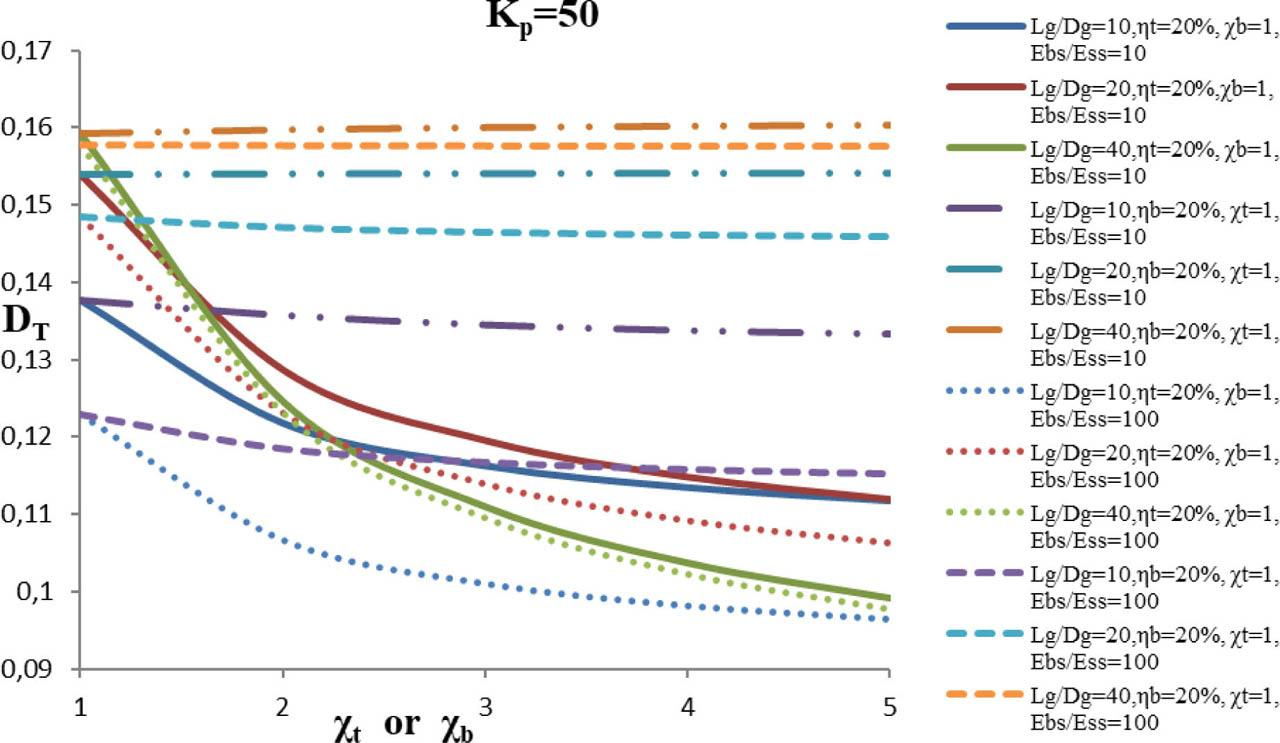Variation of displacement affecting component for the top of GP, DT, with strengthening factor for the top, χt, or strengthening factor for the bottom, χb. Impact of the relative length of GP, Lg/Dg; PLST of GP, ηt; PLSB of GP, ηb; and relative stiffness of bearing stratum, Ebs/Ess, on a single partially strengthened GP (Kp=50, ηt=ηb=20%)

Fig.10 shows the variation of PLTB of GP, (Fb/F)×100 with strengthening factor for the top, χt, or strengthening factor for the bottom, χb. Both the percentage length of strengthening from the top of GP (ηt) and the percentage length of strengthening from the bottom of GP (ηb) are taken as 10% as shown in Fig. 10. It can well be seen from the Fig. 10 that when strengthening effect is only provided at the top of GP, then the curve is almost linear, showing that this is not producing a substantial effect on the parameter of PLTB of GP, (Fb/F)×100. But for the case of strengthening adopted for the bottom, the PLTB of GP, (Fb/F)×100 increases mainly till the strengthening factor for the bottom, χb, becomes 3; thereafter, it becomes constant. Moreover, it can also be seen that the value of PLTB of GP, (Fb/F)×100 increases with an increase in relative stiffness of GP, Kp, and relative stiffness of bearing stratum Ebs/Ess, whatever may be the case, i.e., bottom strengthening or top strengthening. But specifically for the case of bottom stiffening, the PLTB of GP, (Fb/F)×100 increases more for greater value of Ebs/Ess. It may well be observed from the Fig. 10 that at– Lg/Dg=10, Ebs/Ess=100, χt=1, ηb=10%, Kp=100, for χb=1, 2, 3, and 4, the value of PLTB of GP, (Fb/F)×100 are, respectively, 64.74, 68.34, 69.65, and 70.34, thereby causing an increase of 5.5, 7.5, and 8.6 with respect to χb=1.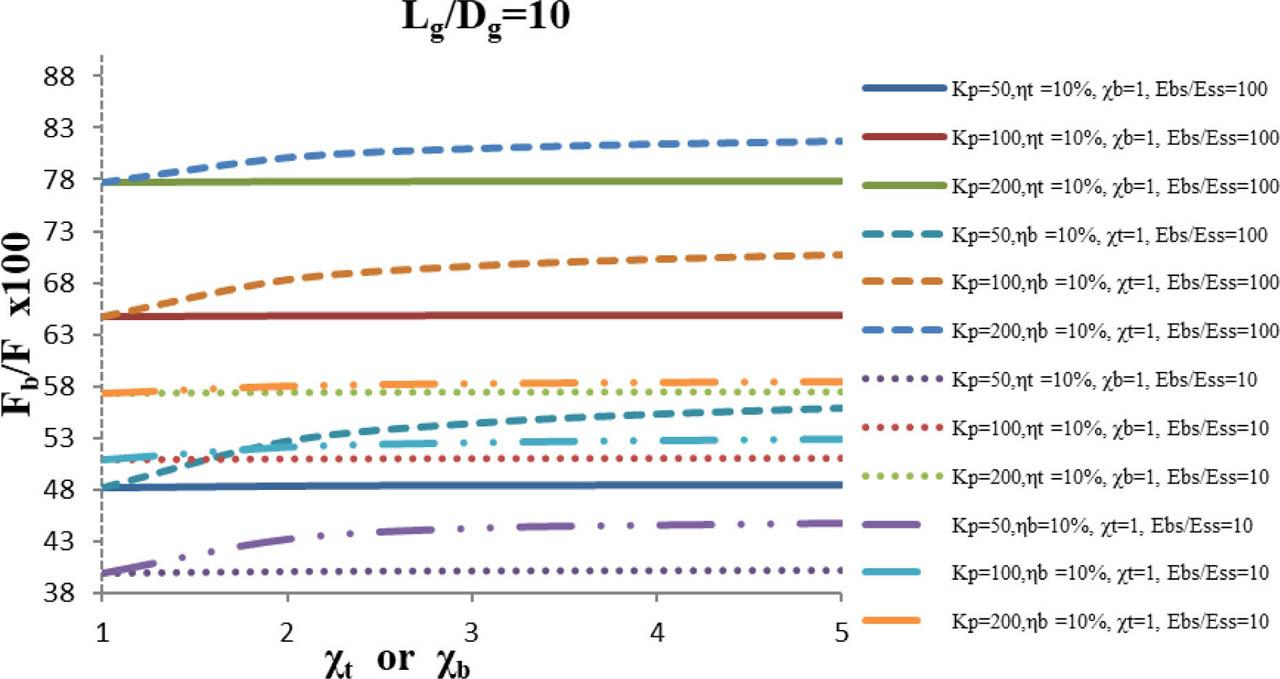Variation of PLTB of GP, (Fb/F)×100 with strengthening factor for the top, χt, or strengthening factor for the bottom, χb. Impact of the relative stiffness of GP, Kp, and relative stiffness of bearing stratum, Ebs/Ess, on a single partially strengthened GP (Lg/Dg=10, ηt or ηb=10%)

Fig. 11 shows the variation of PLTB of GP, (Fb/F)×100 with strengthening factor for the top, χt or strengthening factor for the bottom, χb. Both the percentage length of strengthening from the top of GP (ηt) and the percentage length of strengthening from the bottom of GP (ηb) are further increased in contrast to Fig. 10 and are taken as 20% in Fig. 11. Therefore, it can be observed from the Fig. 11 that the value of PLTB of GP, (Fb/F)×100 is on the higher side as compared to the values in Fig. 10. For instance, from observing the curves it may well be noted that at– Lg/Dg=10, Ebs/Ess=100, χt =1, ηb=20%, Kp=100, for χb=1, 2, 3, and 4, the value of PLTB of GP, (Fb/F)×100 is, respectively, 64.74, 70.37, 72.49, and 73.61, thereby causing a large increase of 8.6, 11.9, and 13.7 with respect to χb=1.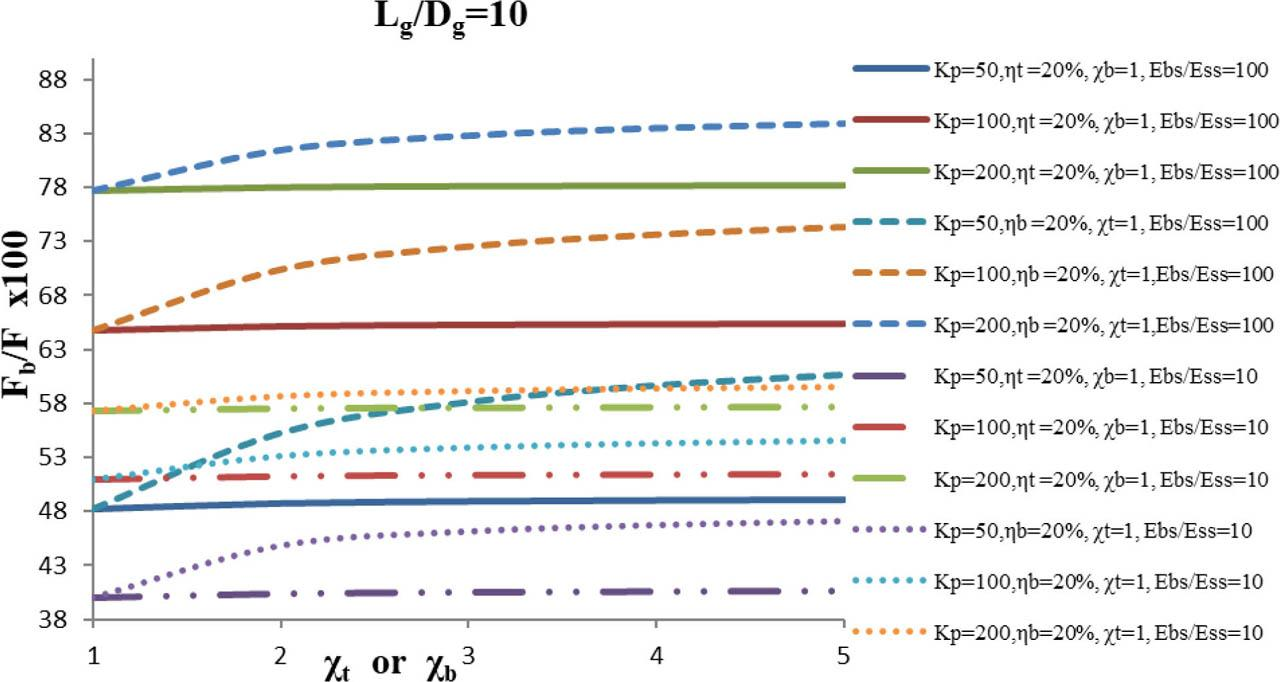Variation of PLTB of GP, (Fb/F) ×100 with strengthening factor for the top, χt, or strengthening factor for the bottom, χb. Impact of relative stiffness of GP, Kp, and relative stiffness of bearing stratum, Ebs/Ess, on a single partially strengthened GP (Lg/Dg=10, ηt or ηb=20%).

Fig. 12 shows the variation of PLTB of GP, (Fb/F)×100 with strengthening factor for the top, χt or strengthening factor for the bottom, χb. Both the PLST of GP and the PLSB of GP are further increased to 30% in the Fig. 12. By increasing the percentage length of strengthening, the values of PLTB of GP increase further. The effect of the relative stiffness of the bearing stratum can well be seen from the curve. As shown in the Fig. 12 the value of PLTB of GP, (Fb/F)×100 at– Lg/Dg=10, Ebs/Ess=100, χt =1, ηb=30%, Kp=100, χb=2 is 72.11%, while with Ebs/Ess=10 and rest parameters as it is, the value turns out to be 53.99%, thereby causing an increase of 33.56% with respect to Ebs/Ess=10.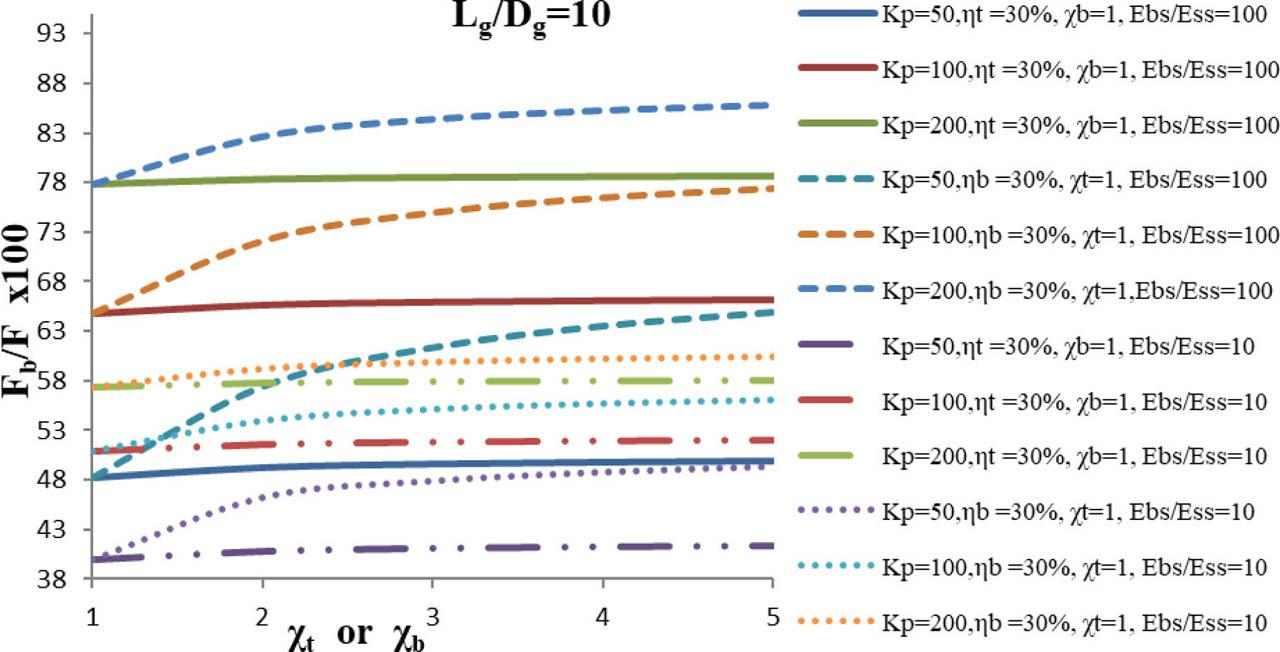Variation of PLTB of GP, (Fb/F)×100 with strengthening factor for the top, χt, or strengthening factor for the bottom, χb. Impact of the relative stiffness of GP, Kp, and relative stiffness of bearing stratum, Ebs/Ess, on a single partially strengthened GP (Lg/Dg=10, ηt or ηb=30%).

Fig. 13 depicts the variation of PLTB of GP, (Fb/F)×100 with strengthening factor for the top, χt, or strengthening factor for the bottom, χb, such that χt= χb. The relative stiffness of GP, Kp, PLST, ηt=10% or 30%, PLSB of GP, ηb=30% and 10% are the parameters which are considered here. Due to the simultaneous effect of the bottom and top strengthening, all the curves in Fig. 13 are curved. Owing to dual strengthening, the values of PLTB of GP, (Fb/F)×100, are certainly higher than values in previous figures. Simultaneously, it can well be concluded from the Fig. 13 that bottom strengthening contributes more for PLTB of GP, (Fb/F)×100. For example it may be seen from the figure that at– Lg/Dg=10, Ebs/Ess=100, ηt=30%, ηb=10%, χt= χb=3 for Kp= 50, 100, 200, and 400, the values of PLTB of GP, (Fb/F)×100 are, respectively, 56.07, 70.94, 81.86, and 88.57, while that at– Lg/Dg=10, Ebs/Ess=100, ηt=10%, ηb=30%, χt= χb=3 for Kp= 50, 100, 200, and 400, the values are, respectively, 61.58, 75.15, 84.48, and 89.87. Thus, it can be seen that the percentage increase in the value of PLTB of GP decreases with the increase in the value of Kp.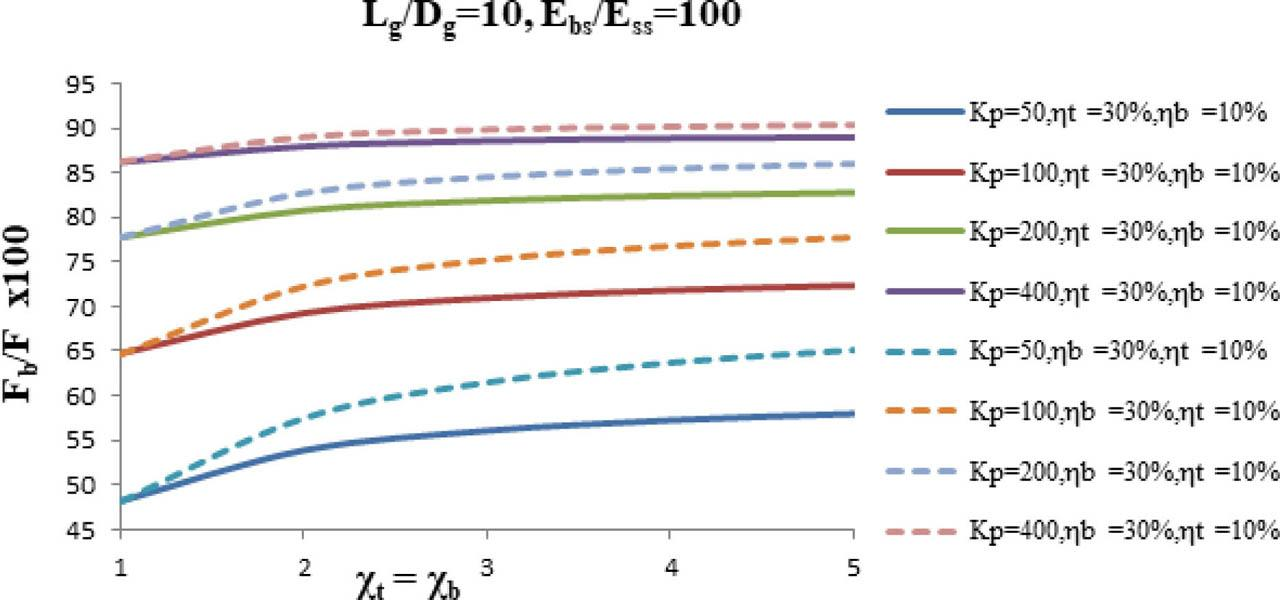Variation of PLTB of GP, (Fb/F)×100 with strengthening factor for the top, χt, or strengthening factor for the bottom, χb, such that χt= χb. Impact of the relative stiffness of GP, Kp; PLST of GP, ηt; PLSB of GP, ηb; on a single partially strengthened GP (Lg/Dg=10, Ebs/Ess=100).

Fig. 14 investigates the impact of relative stiffness of GP, Kp; PLST, ηt; PLSB of GP, ηb; on the variation of PLTB of GP, (Fb/F)×100. The values of both the strengthening factors are kept equal to each other. The PLST of GP, ηt, is taken higher, i.e., 40% and 20%, and similarly PLSB of GP, ηb, varies as 20% and 40%. The trends of the curves are same as earlier; with a difference in values, i.e., in this case the values are slightly higher because of higher percentage length of strengthening. As an example, it may well be established from the Fig. 14 that at– Lg/Dg=10, Ebs/Ess=100, ηt=40%, ηb=20%, χt= χb=3 for Kp= 50, 100, 200, and 400, the values of PLTB of GP, (Fb/F)×100 are, respectively, 61.14, 74.85, 84.33, and 89.85, while that at– Lg/Dg=10, Ebs/Ess=100, ηt=20%, ηb= 40%, χtb=3 for Kp= 50, 100, 200, and 400, the values are, respectively, 65.14, 77.78, 86.09, and 90.67. Thus, it may be concluded that if these data are compared with the data of Fig. 13, then at– Lg/Dg=10, Ebs/Ess=100, ηt= 20%, ηb= 40%, χtb=3 for Kp= 50, 100, 200, and 400, there is an increase in the PLTB of GP, respectively, 5.7, 3.4, 1.9, and 0.8, by increasing top and bottom percent length of strengthening each by 10%, i.e., at– Lg/Dg=10, Ebs/Ess=100, ηt= 10%, ηb= 30%, χtb=3 for Kp= 50, 100, 200, and 400.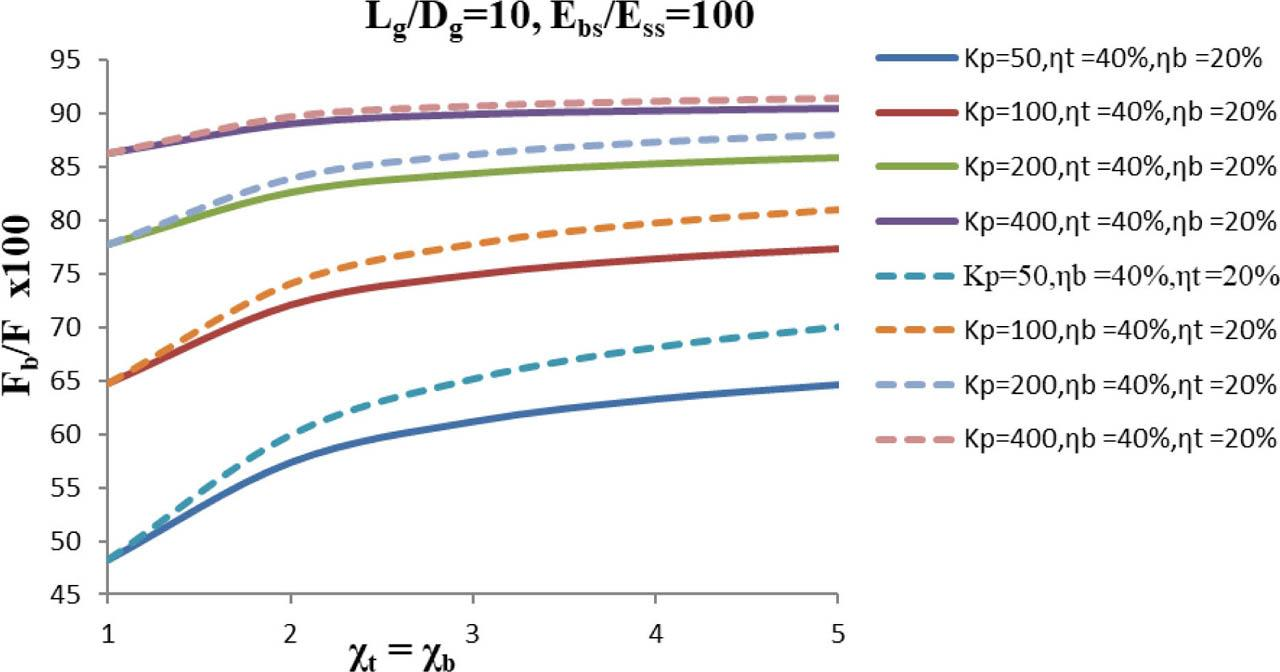Variation of PLTB of GP, (Fb/F)×100 with strengthening factor for the top, χt, or strengthening factor for the bottom, χb, such that χt= χb. Impact of the relative stiffness of GP, Kp; PLST of GP, ηt; PLSB of GP, ηb; on a single partially strengthened GP (Lg/Dg=10, Ebs/Ess=100).

Fig. 15 depicts NSS variation, τ*E=τ(πDgLg)/F with normalized depth, z*g=zg/Lg. The impact of the strengthening factor is shown. In Fig. 15, unstrengthened conditions are depicted, while in other, strengthening effect is presented with strengthening factor at top and bottom. It may well be seen from the Fig. 15 that the NSS is reduced at the top section of GP by introducing the strengthening, and the effect is maximized with the impact of strengthening introduced at both ends. The NSS is redistributed and shifted toward downside of the GP.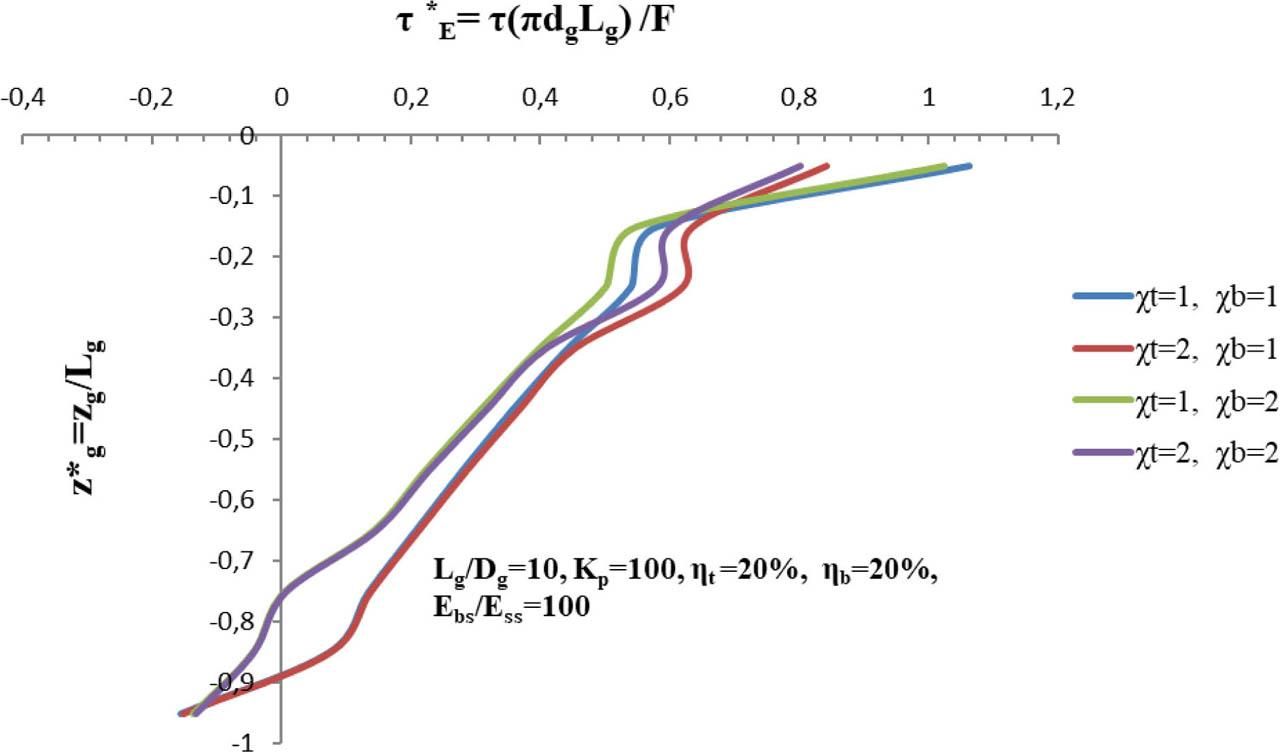Variation of NSS, τ*E= τ(πDgLg)/F with normalized depth, z*g=zg/Lg. Impact of strengthening factor for the top, χt, or strengthening factor for bottom, χb, on a single partially strengthened GP (Lg/Dg=10, Kp=100, ηt=20%, ηb=20%, Ebs/Ess=100).

The variation of NSS, τ*E=τ(πDgLg)/F with normalized depth, z*g=zg/Lg is shown in Fig. 16. The impact of strengthening factor is shown. Since the percent length of strengthening is increased by 10% on both sides, i.e., bottom and top, as compared to Fig. 15, the impact of this change is well visible from the curve that due to bottom strengthening, the NSS is reduced in the bottom strengthened portion of the GP. Rest effects are the same as described earlier.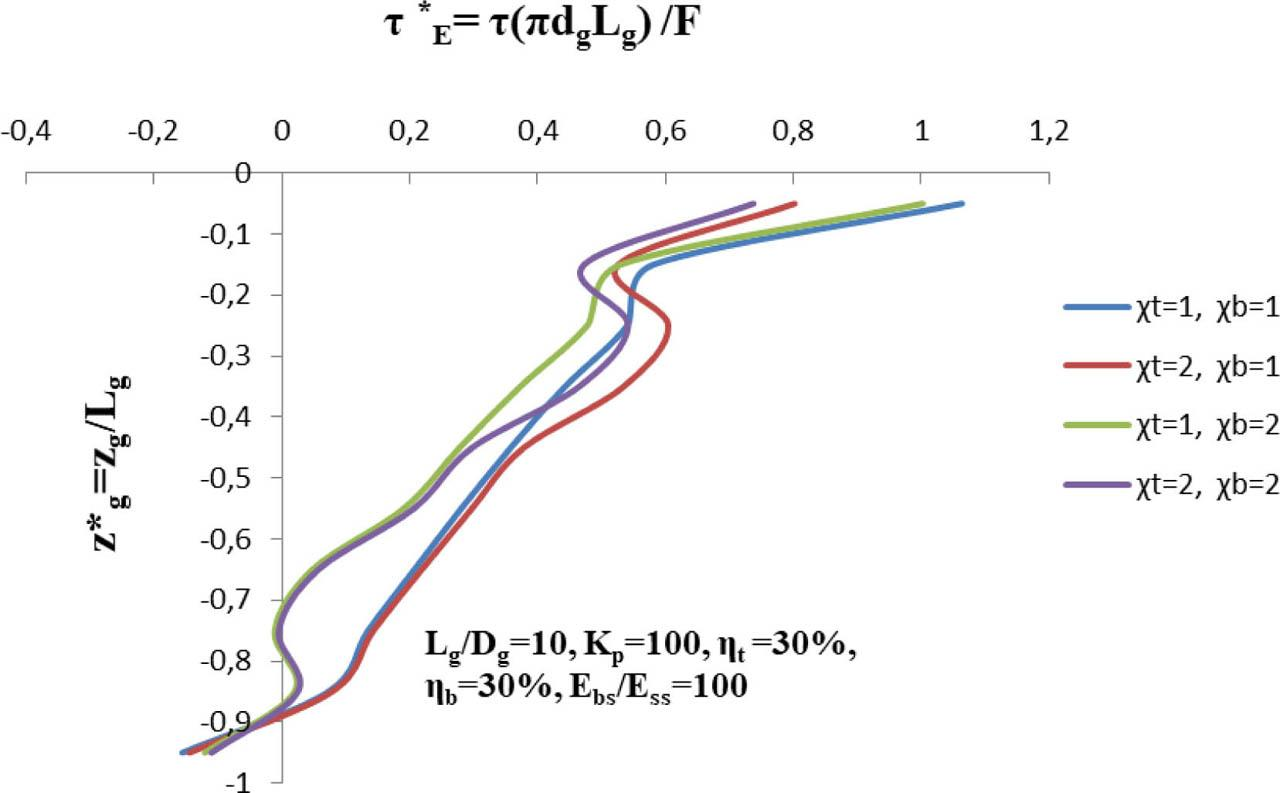Variation of NSS, τ*E= τ(πDgLg)/F with normalized depth, z*g=zg/Lg. Impact of strengthening factor for the top, χt, or strengthening factor for bottom, χb, on a single partially strengthened GP (Lg/Dg=10, Kp=100, ηt=30%, ηb=30%, Ebs/Ess=100).
Conclusions

Partially strengthened single end bearing GP, resting on a bearing stratum, strengthened at both ends is analyzed, using basic mirror image technique and basic integration scheme of Mindlin's equation. An entirely new matrix was developed incorporating the strengthening factor for the top of GP and strengthening factor for the bottom of GP.

It may be concluded that previous studies about the strengthening effect at the top of the GP are more effective in the reduction of displacements at the top, i.e., displacement effecting component for the top of GP as compared to the strengthening effect at the bottom of the GP. As observed from the study, the curve of displacement affecting component for the top of GP versus strengthening factor for either top or bottom reveals that the values of displacement affecting component for the top of GP are reduced more. The figure is a curved one with decreasing trends, in case of strengthening at the top, as in contrast to bottom strengthening, where the curve is almost linear.

In the case of dual strengthening, both the curves for displacement affecting component for the top of GP are curved one with decreasing trends.

It was concluded that as studied earlier, the strengthening at the top is not affecting much the PLTB of GP, but due to bottom strengthening, this value is affected considerably and found to increase. As observed in the analysis, it was noted that by providing strengthening at the top of GP, the PLTB of GP versus strengthening factor curve is almost linear. In contrast, the same figure is curved with increasing values for the case of bottom strengthening.

The PLTB of a strengthened GP is found to increase considerably in the range of strengthening factor for the bottom of GP, χb, varying between 1 and 4. After the value of strengthening factor for the bottom of GP, χb, exceeds 4, the percentage load transferred to the base of the GP increases marginally only.

The study of NSS reveals that due to the dual effect of strengthening, the NSS is reduced at the top of the strengthened GP and is shifted in the downward direction along with the depth of the GP. Similarly, the NSS near the base end is also redistributed.

As for the future scope of the work, the study can be carried out on a group of strengthened end-bearing piles and, a pile with raft system.

#### Validation of results.

Variables verified Lg/Dg Kp and Ebs/Ess Results of Poulos and Mattes  Present analysis with (χtb=1)
DACT (DT) of GP 25 100 and 10 0.54 0.539
25 100 and 100 0.51 0.508
25 100 and 1000 0.49 0.489
20 100 and 100000 0.09 0.0899

Madhav, M. R., Sharma, J. K., & Chandra, S. (2006). Analysis and settlement of a non-homogeneous granular pile. Indian Geotechnical Journal, 36(3), 249–271. MadhavM. R. SharmaJ. K. ChandraS. 2006 Analysis and settlement of a non-homogeneous granular pile Indian Geotechnical Journal 36 3 249 271 Search in Google Scholar

Mattes, N. S., & Poulos, H. G. (1969). Settlement of single compressible pile. Journal of the Soil Mechanics and Foundations Division, 95(1), 189–207. MattesN. S. PoulosH. G. 1969 Settlement of single compressible pile Journal of the Soil Mechanics and Foundations Division 95 1 189 207 Search in Google Scholar

Priebe, H. (1976). Estimating Settlements in a Gravel Column Consolidated Soil. Die Bautechnik 53, 160–163. PriebeH. 1976 Estimating Settlements in a Gravel Column Consolidated Soil Die Bautechnik 53 160 163 Search in Google Scholar

Ambily, A. P., & Gandhi, S. R. (2007). Behavior of stone columns based on experimental and FEM analysis. Journal of geotechnical and geoenvironmental engineering, 133(4), 405–415. AmbilyA. P. GandhiS. R. 2007 Behavior of stone columns based on experimental and FEM analysis Journal of geotechnical and geoenvironmental engineering 133 4 405 415 Search in Google Scholar

Black, J. A., Sivakumar, V., Madhav, M. R., & Hamill, G. A. (2007). Reinforced stone columns in weak deposits: laboratory model study. Journal of Geotechnical and Geoenvironmental Engineering, 133(9), 1154–1161. BlackJ. A. SivakumarV. MadhavM. R. HamillG. A. 2007 Reinforced stone columns in weak deposits: laboratory model study Journal of Geotechnical and Geoenvironmental Engineering 133 9 1154 1161 Search in Google Scholar

Madhav, M. R., Sharma, J. K., & Sivakumar, V. (2009). Settlement of and load distribution in a granular piled raft. Geomechanics and Engineering, 1(1), 97–112. MadhavM. R. SharmaJ. K. SivakumarV. 2009 Settlement of and load distribution in a granular piled raft Geomechanics and Engineering 1 1 97 112 Search in Google Scholar

Wang, G. (2009). Consolidation of soft clay foundations reinforced by stone columns under time-dependent loadings. Journal of geotechnical and geoenvironmental engineering, 135(12), 1922–1931. WangG. 2009 Consolidation of soft clay foundations reinforced by stone columns under time-dependent loadings Journal of geotechnical and geoenvironmental engineering 135 12 1922 1931 Search in Google Scholar

Najjar, S. S., Sadek, S., &Maakaroun, T. (2010). Effect of sand columns on the undrained load response of soft clays. Journal of Geotechnical and Geoenvironmental Engineering, 136(9), 1263–1277. NajjarS. S. SadekS. MaakarounT. 2010 Effect of sand columns on the undrained load response of soft clays Journal of Geotechnical and Geoenvironmental Engineering 136 9 1263 1277 Search in Google Scholar

Black, J. A., Sivakumar, V., & Bell, A. (2011). The settlement performance of stone column foundations. Géotechnique, 61(11), 909–922. BlackJ. A. SivakumarV. BellA. 2011 The settlement performance of stone column foundations Géotechnique 61 11 909 922 Search in Google Scholar

Yoo, C. (2010). Performance of geosynthetic-encased stone columns in embankment construction: numerical investigation. Journal of Geotechnical and Geoenvironmental Engineering, 136(8), 1148–1160. YooC. 2010 Performance of geosynthetic-encased stone columns in embankment construction: numerical investigation Journal of Geotechnical and Geoenvironmental Engineering 136 8 1148 1160 Search in Google Scholar

Shahu, J. T., & Reddy, Y. R. (2011). Clayey soil reinforced with stone column group: model tests and analyses. Journal of Geotechnical and Geoenvironmental Engineering, 137(12), 1265–1274. ShahuJ. T. ReddyY. R. 2011 Clayey soil reinforced with stone column group: model tests and analyses Journal of Geotechnical and Geoenvironmental Engineering 137 12 1265 1274 Search in Google Scholar

Shahu, J. T., & Reddy, Y. R. (2014). Estimating long-term settlement of floating stone column groups. Canadian geotechnical journal, 51(7), 770–781. ShahuJ. T. ReddyY. R. 2014 Estimating long-term settlement of floating stone column groups Canadian geotechnical journal 51 7 770 781 Search in Google Scholar

Etezad, M., Hanna, A. M., &Ayadat, T. (2015). Bearing capacity of a group of stone columns in soft soil. International Journal of Geomechanics, 15(2), 04014043. EtezadM. HannaA. M. AyadatT. 2015 Bearing capacity of a group of stone columns in soft soil International Journal of Geomechanics 15 2 04014043 Search in Google Scholar

Hosseinpour, I., Almeida, M. S. S., & Riccio, M. (2015). Full-scale load test and finite-element analysis of soft ground improved by geotextile-encased granular columns. Geosynthetics International, 22(6), 428–438. HosseinpourI. AlmeidaM. S. S. RiccioM. 2015 Full-scale load test and finite-element analysis of soft ground improved by geotextile-encased granular columns Geosynthetics International 22 6 428 438 Search in Google Scholar

Hong, Y. S., Wu, C. S., & Yu, Y. S. (2016). Model tests on geotextile-encased granular columns under 1-g and undrained conditions. Geotextiles and Geomembranes, 44(1), 13–27. HongY. S. WuC. S. YuY. S. 2016 Model tests on geotextile-encased granular columns under 1-g and undrained conditions Geotextiles and Geomembranes 44 1 13 27 Search in Google Scholar

Garg, V., & Sharma, J. K. (2019). Analysis and settlement of partially stiffened single and group of two floating granular piles. Indian Geotechnical Journal, 49(2), 191–203. GargV. SharmaJ. K. 2019 Analysis and settlement of partially stiffened single and group of two floating granular piles Indian Geotechnical Journal 49 2 191 203 Search in Google Scholar

Madhav, M.R., Sharma, J.K., Garg, Vaibhaw, (2019). Stiffening effect on end bearing granular piles. A special issue Honouring Dr Bengt. Fellenius, “Geotechnical Engineering Journal of The SEAGS and AGSSEA50, no. 3:32–40. MadhavM.R. SharmaJ.K. GargVaibhaw 2019 Stiffening effect on end bearing granular piles. A special issue Honouring Dr Bengt. Fellenius Geotechnical Engineering Journal of The SEAGS and AGSSEA 50 3 32 40 Search in Google Scholar

Mindlin, R. D. (1936). Force at a point in the interior of a semi-infinite solid. physics, 7(5), 195–202. MindlinR. D. 1936 Force at a point in the interior of a semi-infinite solid physics 7 5 195 202 Search in Google Scholar

Mindlin, R. D. (1937). Stress system in a circular disk under radial forces, presented at the joint meeting of applied mechanics and hydraulic division of the ASME held at Cornell University, NY, pp. A115–118. MindlinR. D. 1937 Stress system in a circular disk under radial forces presented at the joint meeting of applied mechanics and hydraulic division of the ASME held at Cornell University NY A115 118 Search in Google Scholar

Poulos, H. G., & Mattes, N. S. (1969). The behaviour of axially loaded end-bearing piles. Geotechnique, 19(2), 285–300. PoulosH. G. MattesN. S. 1969 The behaviour of axially loaded end-bearing piles Geotechnique 19 2 285 300 Search in Google Scholar

Nav, M.A., Rahnavard, R., Noorzad, A. and Napolitano, R., (2020), June. Numerical evaluation of the behavior of ordinary and reinforced stone columns. In Structures (Vol. 25, pp. 481–490). Elsevier. https://doi.org/10.1016/j.istruc.2020.03.021. NavM.A. RahnavardR. NoorzadA. NapolitanoR. 2020 June Numerical evaluation of the behavior of ordinary and reinforced stone columns In Structures 25 481 490 Elsevier https://doi.org/10.1016/j.istruc.2020.03.021. Search in Google Scholar

Szypcio, Z., (2000). Bearing capacity of a single column. Studia Geotechnica et Mechanica, 22(3–4), pp.41–54. SzypcioZ., 2000 Bearing capacity of a single column Studia Geotechnica et Mechanica 22 3–4 41 54 Search in Google Scholar

Sharma, J.K. and Gupta, P., (2018). Analysis and settlement evaluation of an end-bearing granular pile with non-linear deformation modulus. Studia Geotechnica et Mechanica, 40(3), pp.188–201. https://doi.org/10.2478/sgem-2018-0022. SharmaJ.K. GuptaP. 2018 Analysis and settlement evaluation of an end-bearing granular pile with non-linear deformation modulus Studia Geotechnica et Mechanica 40 3 188 201 https://doi.org/10.2478/sgem-2018-0022. Search in Google Scholar

• #### Usefulness of the CPTU method in evaluating shear modulus G0 changes in the subsoil

Recommended articles from Trend MD